# Rbse Solutions for Class 6 maths Chapter 4: Basic Geometrical Ideas

Exercise 4.1 page no: 74 ncert Solutions for Class 6 maths Chapter 4

1. Use the figure to name:

(a) Five points

(b) A line

(c) Four rays

(d) Five line segments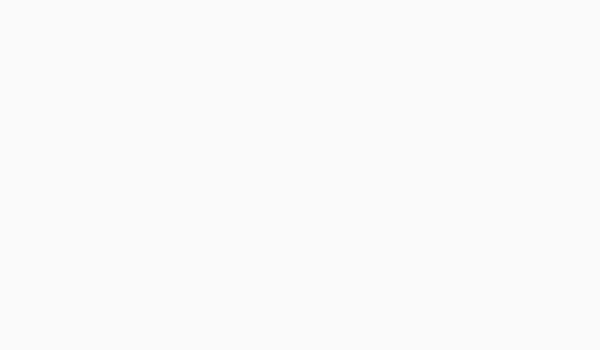ncert Solutions for Class 6 maths Chapter 4

Solutions:

(a) The five points are D, E, O, B and C

(b) A line is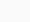(c) Four rays are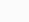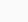and.

(d) Five line segments are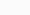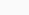and2. Name the line given in all possible (twelve) ways, choosing only two letters at a time from the four given.

Solutions:

The lines are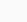3. Use the figure to name:

(a) Line containing point E.

(b) Line passing through A.

(c) Line on which O lies

(d) Two pairs of intersecting lines.ncert Solutions for Class 6 maths Chapter 4

Solutions:

(a) Line containing point E is(b) Line passing through A is(c) Line on which O lies is(d) Two pairs of intersecting lines areand4. How many lines can pass through (a) one given point? (b) two given points?

Solutions:

(a) Countless lines can pass through a given point.

(b) Only one line can pass through a two given points.

5. Draw a rough figure and label suitably in each of the following cases:

(a) Point P lies on .

(b)  and  intersect at M.

(c) Line l contains E and F but not D.

(d)  and  meet at O.

Solutions:

(a)

(b)

(c)

(d)

6. Consider the following figure of line . Say whether following statements are true or false in context of the given figure.

(a) Q, M, O, N, P are points on the line .

(b) M, O, N are points on a line segment .

(c) M and N are end points of line segment.

(d) O and N are end points of line segment .

(e) M is one of the end points of line segment .

(f) M is point on ray .

(g) Rayis different from ray .

(h) Ray  is same as ray .

(i) Ray  is not opposite to ray .

(j) O is not an initial point of

(k) N is the initial point of  and .

Solutions:

(a) True

(b) True

(c) True

(d) False

(e) False

(f) False

(g) True

(h) False

(i) False

(j) False

(k) True

Exercise 4.2 PAGE NO: 78

1. Classify the following curves as (i) Open or (ii) Closed

Solutions:

(a) The given curve is an open curve

(b) The given curve is closed curve

(c) The given curve is open curve

(d) The given curve is closed curve

(e) The given curve is closed curve

2. Draw rough diagrams to illustrate the following:

(a) Open curve

(b) Closed curve

Solutions

(a) The below figure is the open curve

(b) The below figure is the closed curve

3. Draw any polygon and shade its interior.

Solutions:

The below figure is the polygon with interior shade

4. Consider the given figure and answer the questions:

(a) Is it a curve?

(b) Is it closed?

Solutions:

(a) Yes, it is a curve

(b) Yes, it is closed curve

5. Illustrate, if possible, each one of the following with a rough diagram:

(a) A closed curve that is not a polygon.

(b) An open curve made up entirely of line segments.

(c) A polygon with two sides.

Solutions:

(a) The below figure is the closed figure but not a polygon

(b) The below figure is an open curve made up entirely of line segments

(c) No its not possible, as the polygon having least number of sides is a triangle which has three sides.

Exercise 4.3 page no: 80

1. Name the angles in the given figure.

Solutions:

The angles are ∠DAB, ∠ABC, ∠BCD and ∠CDA

2. In the given diagram, name the points(s)

(a) In the interior of ∠DOE

(b) In the exterior of ∠EOF

(c) On ∠EOF

Solutions:

(a) The point in the interior of ∠DOE is A

(b) The point in the exterior of ∠EOF is C, A and D

(c) The points on ∠EOF are E, B, O and F

3. Draw rough diagrams of two angles such that they have

(a) One point in common

(b) Two points in common

(c) Three points in common

(d) Four points in common

(e) One ray in common

Solutions:

(a) O is common point between ∠COD and ∠AOB

(b) O and B are common points between ∠AOB and ∠BOC

(c) O, E and B are common points between ∠AOB and ∠BOC

(d) O, E, D and A are common points between ∠BOA and ∠COA

(e) OC is common ray between ∠BOC and ∠AOC

Exercise 4.4 page no: 81

1. Draw a rough sketch of a triangle ABC. Mark a point P in its interior and a point Q in its exterior. Is the point A in its exterior or in its interior?

Solutions:

Point A lies on the given triangle ABC. It lies neither in interior nor exterior.

2. (a) Identify three triangles in the figure.

(b) Write the names of seven angles.

(c) Write the names of six line segments

(d) Which two triangles have ∠B as common?

Solutions:

(a) The three triangles are ∠ABD, ∠ACB, ∠ADC

(c) The line segments are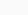(d) ∠ABD and ∠ABC are triangles which have ∠B as common.

Exercise 4.5 Page no: 82

1. Draw a rough sketch of a quadrilateral PQRS. Draw its diagonals. Name them. Is the meeting point of the diagonals in the interior or exterior of the quadrilateral?

Solutions:

PR and QS are the diagonalsThey meet at point O which is in the interior of the quadrilateral.

2. Draw a rough sketch of a quadrilateral KLMN. State,

(a) two pairs of opposite sides,

(b) two pairs of opposite angles,

(c) two pairs of adjacent sides,

(d) two pairs of adjacent angles.

Solutions:

(a) Two pairs of opposite sides are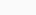and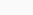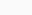(b) Two pairs of opposite angles are ∠KLM, ∠KNM and ∠LKN, ∠LMN

(c) Two pairs of adjacent sides areandorand(d) Two pairs of adjacent angles are ∠K, ∠L and ∠M, ∠N or ∠K, ∠L and ∠L, ∠M

Exercise 4.6 page no: 84

1. From the figure, identify:

(a) the centre of circle

(c) a diameter

(d) a chord

(e) two points in the interior

(f) a point in the exterior

(g) a sector

(h) a segment

Solutions:

(a) The centre of circle is O

(b) Three radii are(c) A diameter is(d) A chord is(e) Two points in the interior are O and P

(f) A point in the exterior is Q

(g) A sector is AOB i.e shaded region

(h) A segment is ED i.e shaded region

2. (a) Is every diameter of a circle also a chord?

(b) Is every chord of a circle also a diameter?

Solutions:

(a) Yes every diameter of a circle is also a chord. Diameter is also called as longest chord.

(b) No, every chord is not a diameter.

3. Draw any circle and mark

(a) its centre

(c) a diameter

(d) a sector

(e) a segment

(f) a point in its interior

(g) a point in its exterior

(h) an arc

Solutions:

(a) The centre of the circle is O.

(b) The radius is OC

(c) A diameter is(d) A sector is AOC

(e) A segment is DE

(f) A point in its interior is O

(g) A point in its exterior is F

(h) An arc is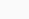4. Say true or false:

(a) Two diameters of a circle will necessarily intersect.

(b) The centre of a circle is always in its interior.

Solutions:

(a) True, two diameters will always intersect each other at the centre of the circle.

(b) True, the centre of the circle will always be in its interior.ncert Solutions for Class 6 maths Chapter 4

Patio umbrellas sold at Costco recalled after reports of fires गर्मी में लू लगने से बचाव करेंगे यह खाद्य पदार्थ, आज ही खाना करें शुरू ट्रेन के बीच में ही AC कोच क्यों लगाए जाते हैं? Rbse books for class 1 to 12 hindi medium 2021-22
ट्रेन के बीच में ही AC कोच क्यों लगाए जाते हैं? गर्मी में लू लगने से बचाव करेंगे यह खाद्य पदार्थ, आज ही खाना करें शुरू Rbse books for class 1 to 12 hindi medium 2021-22 Patio umbrellas sold at Costco recalled after reports of fires